Question 1: ( 1 Marks )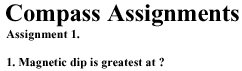A The poles.
 B The equator.

Question 2: ( 1 Marks )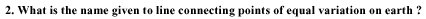A The agonic line.
 B The deviation line.
 C Isotachs.
 D Isogonals

Question 3: ( 1 Marks )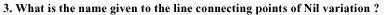A The agonic line.
 B The deviation line.
 C Isotachs.
 D Isogonals

Question 4: ( 1 Marks )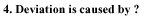A The convergency of the meridians.
 B The convergency of the parallels of latitude.
 C The varying values of the earths variation.
 D The effect of an aircraft’s own magnetic field.

Question 5: ( 1 Marks )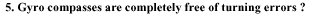A True
 B False

Question 6: ( 1 Marks )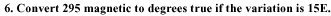A 295T
 B 310T
 C 280T

Question 7: ( 1 Marks )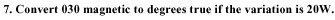A 010T
 B 050T
 C 030T

Question 8: ( 1 Marks )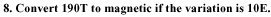A 180M
 B 190M
 C 200M

Question 9: ( 1 Marks )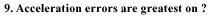Question 10: ( 1 Marks )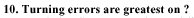Question 11: ( 1 Marks )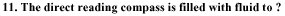A Provide damping and reduce friction.
 B Support the bar magnet.

Question 12: ( 1 Marks )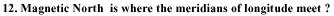A True
 B False

Question 13: ( 1 Marks )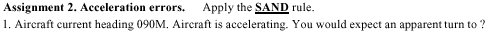A The right
 B The North
 C Decreasing compass numbers.

Question 14: ( 1 Marks )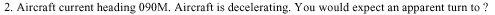A The right
 B The South
 C Decreasing compass numbers.

Question 15: ( 1 Marks )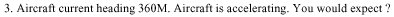A An apparent turn to the right.
 B The South.
 C It to continue reading 360M.

Question 16: ( 1 Marks )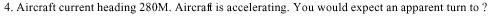A The right
 B The South
 C Increasing compass numbers.

Question 17: ( 1 Marks )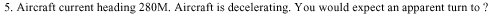A The right
 B The South
 C Decreasing compass numbers.

Question 18: ( 1 Marks )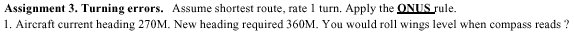A 360M
 B 330M
 C 030M

Question 19: ( 1 Marks )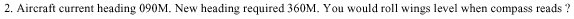A 360M
 B 330M
 C 030M

Question 20: ( 1 Marks )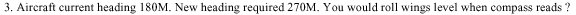A 270M
 B 300M
 C 260M

Question 21: ( 1 Marks )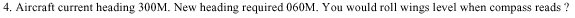A 070M
 B 060M
 C 050M

Question 22: ( 1 Marks )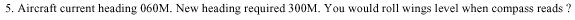A 300M
 B 290M
 C 310M

Question 23: ( 1 Marks )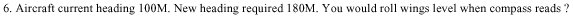A 180M
 B 190M
 C 150M

Question 24: ( 1 Marks )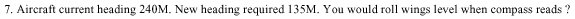A 150M
 B 165M
 C 125M

Press the finish button ONCE only upon completion of the exam